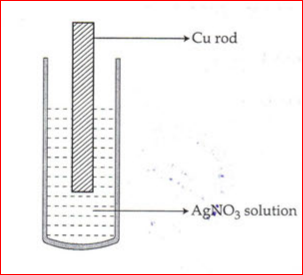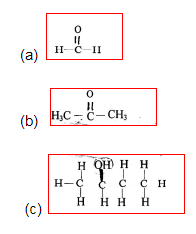# Kerala SSLC Class 10 Chemistry Important Questions

Students who wish to top in the class 10 board exams, need to practice questions. This also helps them in their revision process. Preparing for the exams can be tricky as the student will have to study all the subjects and especially SSLC Chemistry. Students can access the Kerala SSLC Class 10 Chemistry Important Questions and get to practice enough times so that they can easily boost their scores for the board exams very easily.

Other ways to prepare for the exams is to access the SCERT textbooks and other study resources. These help the students to make their exam preparations more effective. Solving these questions will provide students with more confidence to face the exams, as they will be more acquainted with the type of questions that are normally asked at the Kerala SSLC previous year question papers.

### Why Do Students Need to Focus on Kerala Class 10 Chemistry Important Questions?

Most of the previous year question papers are created by subject matter experts on the basis of the SSLC syllabus, so that they focus on important topics of the various subjects. Hence, we at BYJU’s have compiled these important questions on each subject after analyzing the various previous year question papers.

• This gives students an overview of the type of questions
• They are more confident and face the board exams more fearlessly
• Gain more practice
• Self- analyze one’s performance and knowledge gap on a subject
• Prepare better as they are able to plan their study time in a more efficient manner

Know more about the SSLC Class 10 Chemistry Important Questions, here:

1. Which of the following statements is correct about chemical equilibrium
1. At equilibrium both the reactants and products co-exist
2. At equilibrium the rate of the forward reaction is greater than the rate of backward reaction.

2. Observe the diagram below:It shows a copper rod kept immersed in a silver nitrate solution. Find out:

1. What is the colour change of the solution?
2. Write the balanced chemical equation for the reaction.

3. (a) Write an example of a metal that can be refined by liquation.

(b) What is calcination?

4. (a) What are Isomers?

(b) Write the structural formulae of any two position isomers of an alcohol with molecular formula C₅H₁₂O.

5. Organic Compounds are obtained through different chemical reactions.

1. What is the difference between substitution reaction and addition reactions
2. Complete the following reactions:

(i) CH3-CH3+CL2 → _____ + HCL

(ii) CH3 -CH = CH-CH3 +HI → ______

6. Some bacteria obtain their energy by oxidizing sulphur, producing sulphuric acid as a by product. In the laboratory and industrially, the first step in the conversion of Sulphur to Sulphuric Acid is to produce Sulphur dioxide. Then Sulphur dioxide is converted to Sulphur trioxide, which reacts with water producing Sulphuric acid.

1. Name the industrial method of preparing sulphuric acid
2. Name one Catalyst used industrially which speeds up the conversion of Sulphur dioxide to Sulphur trioxide
3. Write the equation for the conversion of Sulphur dioxide to Sulphur trioxide
4. What is the name of the compound created between Sulphur trioxide and sulphuric acid
5. Suggest a method for diluting sulphuric acid

7. State your observations when the following salts are heated. (Equations not needed).

2. Calcium carbonate
3. Zinc carbonate
4. Copper sulphate crystals ( on very strong heating)

8. Write the IUPAC names of the following compounds:9. Calculate the volume occupied by 0.01 mole of carbon dioxide at S.T.P.

10. Draw the electron dot diagram for the following compounds:

1. Methane
2. Calcium Chloride

11. An organic compound whose vapour density is 45, has the following percentage composition. H=2.22%, O=71.19% and rest in Carbon. Calculate its (i) empirical value and (ii) molecular formula.

12. Draw the structural formula of the following compounds:

1. Butan- 2- OL
2. 2,3, dimethyl pentene
3. 2-chloro-4-methyl hexane
4. Pentyne
5. Propanoic acid

13. Arrange the following as per the instruction given in the brackets:

1. H e, Ar, N e (increasing number of the order of electron shells)
2. Na, Li, K(increasing ionization energy)
3. F, Cl, Br (increasing electronegativity)
4. Na, K, Li(increasing atomic size)

14. Match the column A with column B and rewrite the correct pairs:

 COLUMN A COLUMN B (a)Sodium Chloride decreases (b) Ammonium ion Covalent bond (c)Electronegativity in a period Increases (d)Carbon tetrachloride Ionic bond (e) Atomic radii in a group Covalent and coordinate bond

15. Vapour density of a gas Z is 23. Calculate.

(i) Number of moles (ii) weight in grams (iii) number of molecules, in 6.72 litres of gas as S.T.P

Students can also get other resources to prepare for the exams. To know more about Kerala SSLC Chemistry and study materials, stay tuned to BYJU’s.